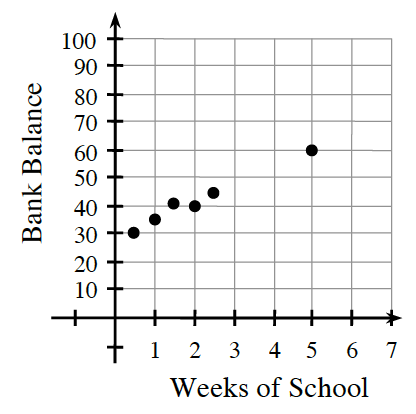Home > CC3 > Chapter 8 > Lesson 8.2.4 > Problem8-113

8-113.

Since the beginning of school, Steven has been saving money to buy a new MP3 player. His bank balance is represented by the graph below.

1. According to the graph, about how much money had Steven saved after $2$ weeks of school?

Since the $x$-axis represents the number of weeks of school, locate $x=2$ and move vertically along the line until you find the graphed point. What is the $y$-coordinate for this point?

He's saved about $40.$

2. About how much money did Steven probably have after $4$ weeks of school? How can you tell?

Imagine a line that best represents the trend of the graph.

What is the $y$-coordinate of the line when $x$ is $4$?

about $55$

3. If he keeps saving at the same rate, how much will he have saved by Week 7? Explain how you know.

Follow the steps in part (b).